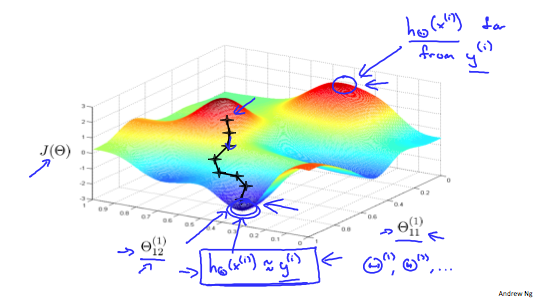# 神经网络

### 逻辑回归的问题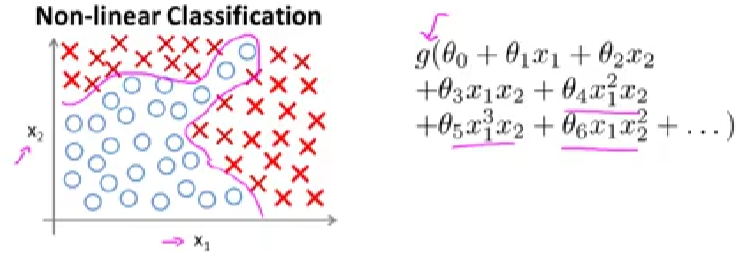• 构建二阶多项式
• 如$x_1^{2}$,$2x_1$,$3x_1$,…,$x_2^{2}$,$3x_2$…有约为5000项(n^2/2)，计算的代价非常高。
• 取一个子集，比如x1^2, x2^2,x3^2...x100^2，这样就只有100个feature，但是100个feature会导致结果误差很高
• 构建三阶多项式
• x1x2x3, x1^2x2, x1^2x3...x10x11x17..., 有约为n^3量级的组合情况，约为170,000个### 单层神经网络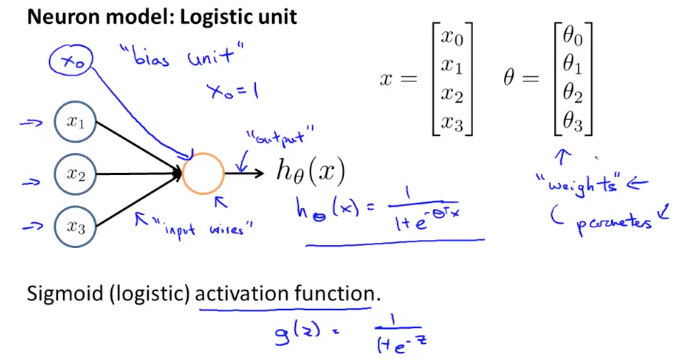• 输入端$x_0$默认为1，也叫做”bias unit.”
• $\theta$矩阵在神经网络里也被叫做权重weight矩阵

import torch

def activation(x):
return 1 / (1+torch.exp(-x))

## Generate some data
torch.manual_seed(7) #set the random seed so things are predictable

## Features are 5 random normal variables
features = torch.randn((1,5)) #1x5

# True weights for our data, random normal variables again
# same shape as features
weights = torch.randn_like(features) #1x5

#and a true bias term
bias = torch.randn((1,1))

# weights.view will convert the matrix to 5x1
# torch.mm does the matrxi multiplication
y = activation(bias + torch.mm(features, weights.view(5,1)))


### 多层神经网络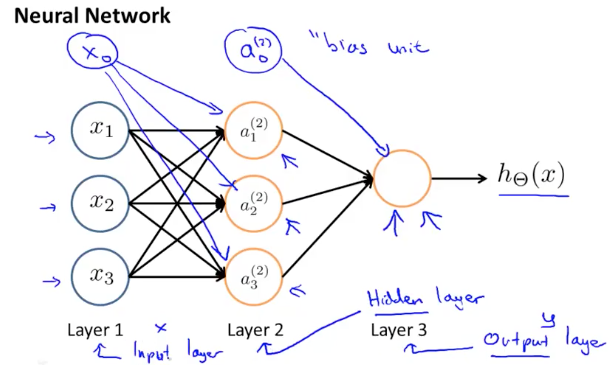• 输入层的$x_i$为样本feature，$x_0$作为”bias”
• 中间层的$a_i^{(j)}$ 表示第j层的第i个节点，我们可以加入$a_0^{(2)}$作为”bias unit”，也可以忽略

${z}_{k}^{\left(2\right)}={\mathrm{\Theta }}_{k,0}^{\left(1\right)}{x}_{0}+{\mathrm{\Theta }}_{k,1}^{\left(1\right)}{x}_{1}+\cdots +{\mathrm{\Theta }}_{k,n}^{\left(1\right)}{x}_{n}$

$\begin{array}{rl}x=\left[\begin{array}{c}{x}_{0}\\ {x}_{1}\\ \cdots \\ {x}_{n}\end{array}\right]& {z}^{\left(j\right)}=\left[\begin{array}{c}{z}_{1}^{\left(j\right)}\\ {z}_{2}^{\left(j\right)}\\ \cdots \\ {z}_{n}^{\left(j\right)}\end{array}\right]\end{array}$

${z}^{\left(j\right)}={\mathrm{\Theta }}^{\left(j-1\right)}{a}^{\left(j-1\right)}$

${a}^{\left(j\right)}=g\left({z}^{\left(j\right)}\right)$

${z}^{\left(j+1\right)}={\mathrm{\Theta }}^{\left(j\right)}{a}^{\left(j\right)}$

${h}_{\mathrm{\Theta }}\left(x\right)={a}^{\left(j+1\right)}=g\left({z}^{\left(j+1\right)}\right)$


function g = sigmoid(z)
g = 1.0 ./ (1.0 + exp(-z));
end

function p = predict(Theta1, Theta2, X)

m = size(X, 1);
num_labels = size(Theta2, 1);

% You need to return the following variables correctly
p = zeros(size(X, 1), 1);

a1 = [ones(m, 1), X];
a2 = sigmoid(a1*Theta1');
a2 = [ones(m,1) a2];
h = sigmoid(a2*Theta2');

% Hint: The max function might come in useful. In particular, the max
%       function can also return the index of the max element, for more
%       information see 'help max'. If your examples are in rows, then, you
%       can use max(A, [], 2) to obtain the max for each row.
%
[max,index] = max(h,[],2);
p = index;

end



### Neural Network Example

• 单层神经网络实现与或门

$\begin{array}{r}\left[\begin{array}{c}{x}_{0}\\ {x}_{1}\\ {x}_{2}\end{array}\right]\to \left[\begin{array}{c}g\left({z}^{\left(2\right)}\right)\end{array}\right]\to {h}_{\mathrm{\Theta }}\left(x\right)\end{array}$ ${x}_{0}$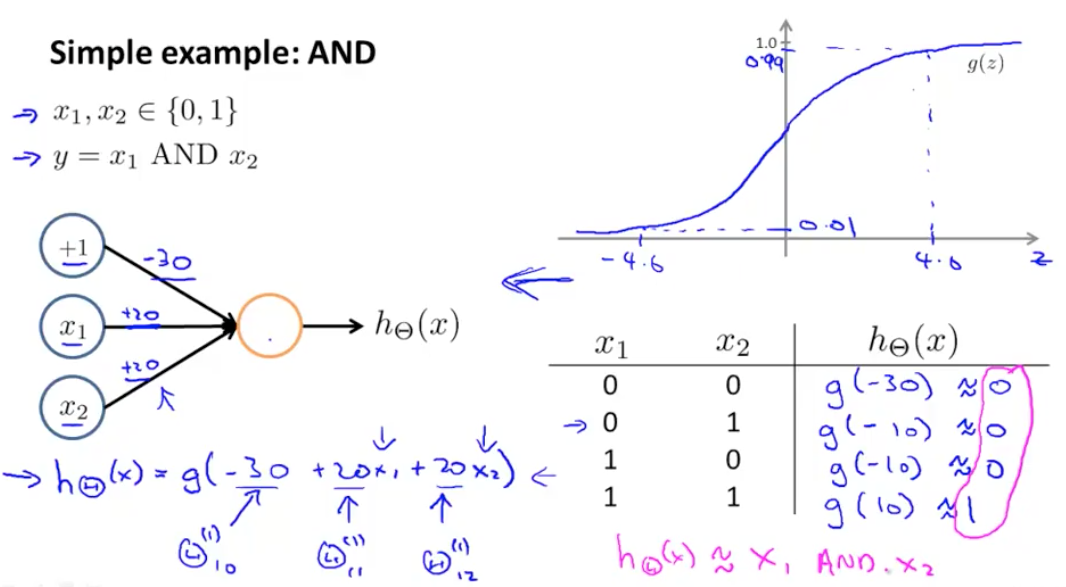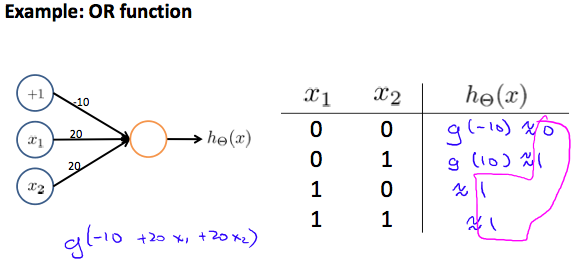• 二级神经网络构建同或门

</br>

$\begin{array}{r}AND:\\ {\mathrm{\Theta }}^{\left(1\right)}& =\left[\begin{array}{ccc}-30& 20& 20\end{array}\right]\\ NOR:\\ {\mathrm{\Theta }}^{\left(1\right)}& =\left[\begin{array}{ccc}10& -20& -20\end{array}\right]\\ OR:\\ {\mathrm{\Theta }}^{\left(1\right)}& =\left[\begin{array}{ccc}-10& 20& 20\end{array}\right]\end{array}$

$\begin{array}{r}\left[\begin{array}{c}{x}_{0}\\ {x}_{1}\\ {x}_{2}\end{array}\right]\to \left[\begin{array}{c}{a}_{1}^{\left(2\right)}\\ {a}_{2}^{\left(2\right)}\end{array}\right]\to \left[\begin{array}{c}{a}^{\left(3\right)}\end{array}\right]\to {h}_{\mathrm{\Theta }}\left(x\right)\end{array}$

${\mathrm{\Theta }}^{\left(1\right)}=\left[\begin{array}{ccc}-30& 20& 20\\ 10& -20& -20\end{array}\right]$

${\mathrm{\Theta }}^{\left(2\right)}=\left[\begin{array}{ccc}-10& 20& 20\end{array}\right]$

$\begin{array}{rl}& {a}^{\left(2\right)}=g\left({\mathrm{\Theta }}^{\left(1\right)}\cdot x\right)\\ & {a}^{\left(3\right)}=g\left({\mathrm{\Theta }}^{\left(2\right)}\cdot {a}^{\left(2\right)}\right)\\ & {h}_{\mathrm{\Theta }}\left(x\right)={a}^{\left(3\right)}\end{array}$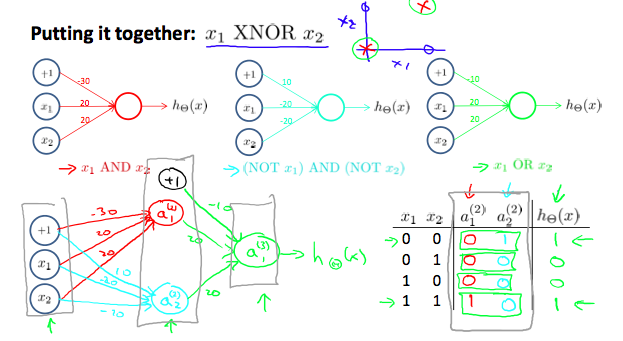### Multiclass Classification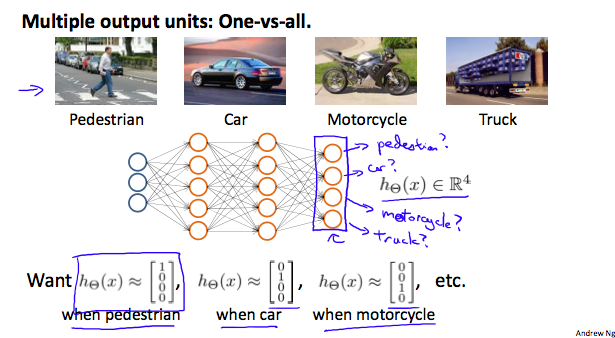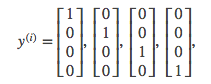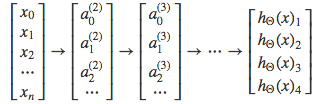### Cost Function

• 先定义一些变量:
• L = 神经网络的层数
• ${S}_{l}$

= 第l层的节点数

• K = 输出层的节点数，即输出结果的种类。
• 对0和1的场景，K=1， ${S}_{l}=1$
• 对于多种分类的场景，K>=3， ${S}_{l}=K$
• ${h}_{\Theta }\left(x{\right)}_{k}$表示第K个分类的计算结果
• Cost Function

$J ( Θ ) = − 1 m ∑ i = 1 m ∑ k = 1 K y k ( i ) log ⁡ ( ( h Θ ( x ( i ) ) ) k ) + ( 1 − y k ( i ) ) log ⁡ ( 1 − ( h Θ ( x ( i ) ) ) k ) + λ 2 m ∑ l = 1 L − 1 ∑ i = 1 s l ∑ j = 1 s l + 1 ( Θ j , i ( l ) ) 2$

1. 前两个求和符号是对每层神经网络节点进行逻辑回归cost function运算后求和
2. 后面三个求和符号是是每层神经网络节点的θ矩阵平方和的累加求和
3. 特殊注意的是，后面三个求和符号中第一个求和符号中的i代表层数的index，不代表训练样本的index
• Octave demo


function [J grad] = nnCostFunction(num_labels,X, y, Theta1, Theta2, lambda)

% X:5000x400
% y:5000x1
% num_labels:10
% Theta1: 25x401
% Theta2: 10x26

% Setup some useful variables
m = size(X, 1);
J = 0;

% make Y: 5000x10
I = eye(num_labels);
Y = zeros(m, num_labels);
for i=1:m
Y(i, :)= I(y(i), :);
end

% cost function
J = (1/m)*sum(sum((-Y).*log(h) - (1-Y).*log(1-h), 2));
% regularization item
r = (lambda/(2*m))*(sum(sum(Theta1(:, 2:end).^2, 2)) + sum(sum(Theta2(:,2:end).^2, 2)));

J = J+r;

end


## Backpropagation algotrithm

“Backpropagation”是神经网络用来求解Cost Function最小值的算法，类似之前线性回归和逻辑回归中的梯度下降法。上一节我们已经了解了Cost Function的定义，我们的目标是求解：

$\underset{\mathrm{\Theta }}{min}J\left(\mathrm{\Theta }\right)$

$\frac{\mathrm{\partial }}{\mathrm{\partial }{\mathrm{\Theta }}_{i,j}^{\left(l\right)}}J\left(\mathrm{\Theta }\right)$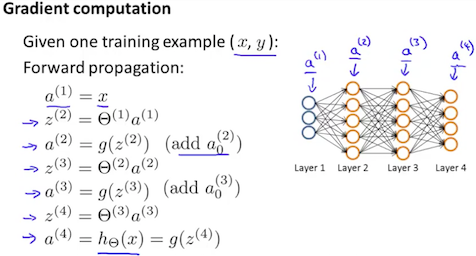$δj(l)="error" of node j in layer l$

$δj(4)=aj(4)-yj$

$δ(4)=a(4)-y$

</br> 第一层是输入层，是样本数据，没有错误，因此不存在${\delta }^{\mathrm{\left(1\right)}}$
</br>

$∂∂Θi,j(l)J(Θ)=aj(l)δi(l+1)$

• 对所有$i,j,l$ ，令 ${\mathrm{\Delta }}_{i,j}^{\left(l\right)}$ = 0，得到一个全零矩阵

• For i=1 to m 做循环，每个循环体执行下面操作

1. ${a}^{\left(1\right)}:={x}^{\left(t\right)}$

让神经网络第一层等于输入的训练数据

2. ${a}^{\left(l\right)}$ 进行“Forward Propagation”计算，其中 l=2,3,…,L 计算过程如上文图示

3. 使用 ${y}^{\left(t\right)}$来计算 ${\delta }^{\mathrm{\left(L\right)}}={a}^{\mathrm{\left(L\right)}}-{y}^{\left(t\right)}$

4. 根据 ${\delta }^{\mathrm{\left(L\right)}}$ 向前计算 ${\delta }^{\left(L-1\right)},{\delta }^{\left(L-2\right)},\dots ,{\delta }^{\left(2\right)}$，公式为： 这个过程涉及到了链式法则，在下一节会介绍

5. ${\mathrm{\Delta }}_{i,j}^{\left(l\right)}:={\mathrm{\Delta }}_{i,j}^{\left(l\right)}+{a}_{j}^{\left(l\right)}{\delta }_{i}^{\left(l+1\right)}$

对每层的θ矩阵偏导不断叠加，进行梯度下降，前面的式子也可以用向量化表示 ${\mathrm{\Delta }}^{\left(l\right)}:={\mathrm{\Delta }}^{\left(l\right)}+{\delta }^{\left(l+1\right)}\left({a}^{\left(l\right)}{\right)}^{T}$

• 加上Regularization项得到最终的θ矩阵

• 当 j≠0 时，$D_{i,j}^{(l)} \thinspace := \thinspace \frac{1}{m}(\Delta_{i,j}^{(l)} + \lambda \Theta_{i,j}^{(l)}), \thinspace if \thinspace$
• 当 j=0 时，$D_{i,j}^{(l)} \thinspace := \thinspace \frac{1}{m}\Delta_{i,j}^{(l)}$

### Backpropagation Intuition$h Θ ( x ) = a 1 ( 3 ) = g ( Θ 10 ( 2 ) a 0 ( 2 ) + Θ 11 ( 2 ) a 1 ( 2 ) + Θ 12 ( 2 ) a 2 ( 2 ) + Θ 13 ( 2 ) a 3 ( 2 ) )$

1. ${h}_{\mathrm{\Theta }}\left(x\right)$

是以Θ为变量的函数

2. 它的计算过程是从第一层开始向最后一层逐层计算，每一层每个节点的值是由它后一层的节点乘以权重矩阵Θ

BP的计算和推导不如Forward容易理解，也不直观，它的特点类和FB类似

1. δ也是自变量为θ的函数
2. 它的计算过程是从最后一层开始向第一层逐层计算，每层的δ值是由它前面一层的δ值乘以权重矩阵θ
3. 它的计算包含两部分，第一部分是求梯度（对θ求偏导），第二部分是梯度下降

$J\left(\mathrm{\Theta }\right)\approx \left({h}_{\mathrm{\Theta }}\left({x}^{\left(i\right)}\right)-{y}^{\left(i\right)}{\right)}^{2}$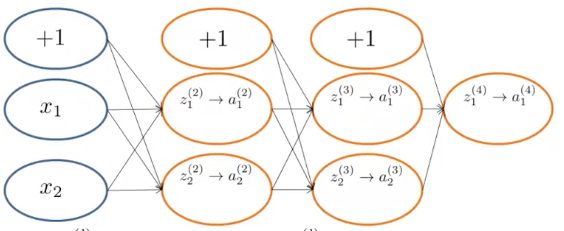• ${x}_{1}$${x}_{2}$ 表示神经网络的输入样本，两个特征
• ${z}_{j}^{\mathrm{\left(l\right)}}$表示第l层的第j个节点的输入值
• ${a}_{j}^{\mathrm{\left(l\right)}}$表示第l层的第j个节点的输出值
• ${\Theta }_{ji}^{\mathrm{\left(l\right)}}$表示第l层到第l+1层的权重矩阵
• ${\delta }_{j}^{\left(l\right)}$表示第l层第j个节点的预测偏差值，他的数学定义为${\delta }_{j}^{\mathrm{\left(l\right)}}=\frac{\mathrm{\partial }}{\mathrm{\partial }{z}_{j}^{\mathrm{\left(l\right)}}}J\left(\mathrm{\Theta }\right)$

1. 参考上面几节，我们令${h}_{\Theta }\left({x}^{\left(t\right)}\right)=g\left({z}^{\left(t\right)}\right)={a}^{\mathrm{\left(t\right)}}$，其中$g$为sigmoid函数$g\mathrm{\left(z\right)}=\frac{1}{1+{e}^{-z}}$

2. 先求 ${\Theta }_{11}^{\mathrm{\left(3\right)}}$，由链式规则，可以做如下运算：$\frac{\partial J\left(\Theta \right)}{\mathrm{\partial }{\Theta }_{11}^{\left(3\right)}}=\frac{\mathrm{\partial }J\left(\mathrm{\Theta }\right)}{\mathrm{\partial }{a}_{1}^{\mathrm{\left(4\right)}}}\ast \frac{\mathrm{\partial }{a}_{1}^{\left(4\right)}}{\mathrm{\partial }{z}_{1}^{\left(4\right)}}\ast \frac{\mathrm{\partial }{z}_{1}^{\left(4\right)}}{\mathrm{\partial }{\mathrm{\Theta }}_{11}^{\left(3\right)}}$

3. 参考上面δ的定义，可知上面等式后两项为：${\delta }_{1}^{\mathrm{\left(4\right)}}=\frac{\mathrm{\partial }J\left(\mathrm{\Theta }\right)}{\mathrm{\partial }{a}_{1}^{\mathrm{\left(4\right)}}}\ast \frac{\mathrm{\partial }{a}_{1}^{\left(4\right)}}{\mathrm{\partial }{z}_{1}^{\left(4\right)}}$， 即输出层第一个节点的误差值，展开计算如下：

$δ1(4)= ∂ J ( Θ ) ∂ a 1 (4) ∗ ∂ a 1 ( 4 ) ∂ z 1 ( 4 ) =− [ y ∗ ( 1 − g ( z ) ) + ( y − 1 ) ∗ g ( z ) ]= − [ y ∗ ( 1 − g ( z ) ) + ( y − 1 ) ∗ g ( z ) ] = g ( z ) − y = a ( 4 ) − y$

，其中用到了sigmoid函数一个特性：$g\mathrm{\prime }\left(z\right)=g\left(z\right)\ast \left(1-g\left(z\right)\right)$

4. 这样我们得到了${\delta }_{1}^{\mathrm{\left(4\right)}}$（参考上一节BP算法的步骤(3)），接下来继续求解$\frac{\partial J\left(\Theta \right)}{\partial {\mathrm{\Theta }}_{11}^{\left(3\right)}}$，前面第二步等号后的最有一项$\frac{\mathrm{\partial }{z}_{1}^{\left(4\right)}}{\mathrm{\partial }{\mathrm{\Theta }}_{11}^{\left(3\right)}}$，将${z}_{1}^{\left(4\right)}$展开有：${z}_{1}^{\left(4\right)}={\Theta }_{10}^{\mathrm{\left(3\right)}}*{a}_{0}^{\mathrm{\left(3\right)}}+{\Theta }_{11}^{\mathrm{\left(3\right)}}*{a}_{1}^{\mathrm{\left(3\right)}}+{\Theta }_{12}^{\mathrm{\left(3\right)}}*{a}_{2}^{\mathrm{\left(3\right)}}$，对${\Theta }_{11}^{\mathrm{\left(3\right)}}$求偏导的结果为${a}_{1}^{\mathrm{\left(3\right)}}$

5. 将第4步与第三步的式子合并，即得出 $\frac{\partial J\left(\Theta \right)}{\partial {\mathrm{\Theta }}_{11}^{\left(3\right)}}={\delta }_{1}^{\mathrm{\left(4\right)}}*{a}_{1}^{\mathrm{\left(3\right)}}$ 与上一节BP算法步骤(5)一致

6. 接下来计算${\Theta }_{11}^{\mathrm{\left(2\right)}}$，链式规则可做如下运算$\frac{\partial J\left(\Theta \right)}{\mathrm{\partial }{\Theta }_{11}^{\left(2\right)}}=\frac{\mathrm{\partial }J\left(\mathrm{\Theta }\right)}{\mathrm{\partial }{a}_{1}^{\mathrm{\left(4\right)}}}\ast \frac{\mathrm{\partial }{a}_{1}^{\left(4\right)}}{\mathrm{\partial }{z}_{1}^{\left(4\right)}}\ast \frac{\partial {z}_{1}^{\left(4\right)}}{\partial {a}_{1}^{\left(3\right)}}*\frac{\partial {a}_{1}^{\left(3\right)}}{\partial {z}_{1}^{\left(3\right)}}*\frac{\mathrm{\partial }{z}_{1}^{\left(3\right)}}{\mathrm{\partial }{\mathrm{\Theta }}_{11}^{\left(2\right)}}$

7. 参考上面δ的定义，可知${\delta }_{1}^{\left(3\right)}=\frac{\mathrm{\partial }J\left(\mathrm{\Theta }\right)}{\mathrm{\partial }{a}_{1}^{\mathrm{\left(4\right)}}}\ast \frac{\mathrm{\partial }{a}_{1}^{\left(4\right)}}{\mathrm{\partial }{z}_{1}^{\left(4\right)}}\ast \frac{\partial {z}_{1}^{\left(4\right)}}{\partial {a}_{1}^{\left(3\right)}}*\frac{\partial {a}_{1}^{\left(3\right)}}{\partial {z}_{1}^{\left(3\right)}}$，由上面的步骤3可知，等式的前两项为${\delta }_{1}^{\left(4\right)}$。这里可以看出对δ值的计算和之前的FB算法类似，如果将神经网络反向来看，当前层的${\delta }^{1}$值是根据后一层的${\delta }^{\mathrm{\left(1-1\right)}}$计算得来。等式的第三项，将${z}_{1}^{\mathrm{\left(4\right)}}$展开后对${a}_{1}^{\mathrm{\left(3\right)}}$求导后得到${\Theta }_{11}^{\mathrm{\left(3\right)}}$，等式最后一项为$g\mathrm{\prime }\left({z}_{1}^{\left(3\right)}\right)$

8. 将上一步的结果进行整理得到: ${\delta }_{1}^{\left(3\right)}={\delta }_{1}^{\left(4\right)}*{\Theta }_{11}^{\mathrm{\left(3\right)}}*g\mathrm{\prime }\left({z}_{1}^{\left(3\right)}\right)$ 和上一节BP算步骤(4)一致

9. 将8的结果带入第6步，可得出$\frac{\partial J\left(\Theta \right)}{\mathrm{\partial }{\Theta }_{11}^{\left(2\right)}}={\delta }_{1}^{\left(3\right)}*\frac{\mathrm{\partial }{z}_{1}^{\left(3\right)}}{\mathrm{\partial }{\mathrm{\Theta }}_{11}^{\left(2\right)}}$，将${z}_{1}^{\mathrm{\left(3\right)}}$展开后对${\mathrm{\Theta }}_{11}^{\left(2\right)}$求导得到${a}_{1}^{\mathrm{\left(2\right)}}$

10. 整理第9步结果可知 $\frac{\partial J\left(\Theta \right)}{\mathrm{\partial }{\Theta }_{11}^{\left(2\right)}}={\delta }_{1}^{\left(3\right)}*{a}_{1}^{\mathrm{\left(2\right)}}$，与上一节步骤（5）一致

$∂∂Θi,j(l)J(Θ)=aj(l)δi(l+1)$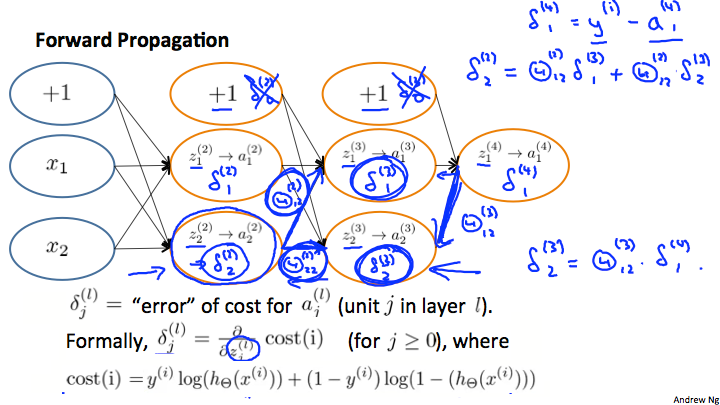1. 自输出层向输入层（即反向传播），逐层求偏导，在这个过程中逐渐得到各个层的参数梯度。

2. 在反向传播过程中，使用 δ(l)δ(l) 保存了部分结果，避免了大量的重复运算，因而该算法性能优异。

### Implementation Nodte: Unrolling parameters

function [jVal, gradient] = costFunction(theta)

...

optTheta = fminunc(@costFunction, initialTheta, options)



fminunc的第二个参数initialTheta需要传入一个vector，而我们之前推导的神经网络权重矩阵Θ显然不是一维的向量，对于一个四层的神经网络来说：

• Θ矩阵：${\Theta }^{\mathrm{\left(1\right)}}$${\Theta }^{\mathrm{\left(2\right)}}$${\Theta }^{\mathrm{\left(3\right)}}$ - matrices(Theta1, Theta2, Theta3)
• 梯度矩阵：${D}^{\mathrm{\left(1\right)}}$${D}^{\mathrm{\left(2\right)}}$${D}^{\mathrm{\left(3\right)}}$ - matrices(D1, D2, D3)

thetaVector = [ Theta1(:); Theta2(:); Theta3(:); ]
deltaVector = [ D1(:); D2(:); D3(:) ]



Theta1 = reshape(thetaVector(1:110),10,11)
Theta2 = reshape(thetaVector(111:220),10,11)
Theta3 = reshape(thetaVector(221:231),1,11)


• 前面得到的thetaVector代入到fminunc中，替换initialTheta
• costFunction中，输入的参数是thetaVec

  function[jVal,gradientVec] = costFunction(thetaVec)


costFunction中，我们需要使用reshape命令从theVec取出${\Theta }^{\mathrm{\left(1\right)}}$${\Theta }^{\mathrm{\left(2\right)}}$${\Theta }^{\mathrm{\left(3\right)}}$ 用来计算FB和BP算法，得到${D}^{\mathrm{\left(1\right)}}$${D}^{\mathrm{\left(2\right)}}$${D}^{\mathrm{\left(3\right)}}$ 梯度矩阵和$J\mathrm{\left(\Theta \right)}$，然后再unroll ${D}^{\mathrm{\left(1\right)}}$${D}^{\mathrm{\left(2\right)}}$${D}^{\mathrm{\left(3\right)}}$得到gradientVec

$\frac{\mathrm{\partial }}{\mathrm{\partial }\mathrm{\Theta }}J\left(\mathrm{\Theta }\right)\approx \frac{J\left(\mathrm{\Theta }+ϵ\right)-J\left(\mathrm{\Theta }-ϵ\right)}{2ϵ}$

$\frac{\mathrm{\partial }}{\mathrm{\partial }{\mathrm{\Theta }}_{j}}J\left(\mathrm{\Theta }\right)\approx \frac{J\left({\mathrm{\Theta }}_{1},\dots ,{\mathrm{\Theta }}_{j}+ϵ,\dots ,{\mathrm{\Theta }}_{n}\right)-J\left({\mathrm{\Theta }}_{1},\dots ,{\mathrm{\Theta }}_{j}-ϵ,\dots ,{\mathrm{\Theta }}_{n}\right)}{2ϵ}$

epsilon = 1e-4;
for i = 1:n,
thetaPlus = theta;
thetaPlus(i) += epsilon;
thetaMinus = theta;
thetaMinus(i) -= epsilon;
end;


1. 通过实现BP算法得到δ矩阵DVec（Unrolled ${D}^{\mathrm{\left(1\right)}}$${D}^{\mathrm{\left(2\right)}}$${D}^{\mathrm{\left(3\right)}}$
2. 进行梯度检查，计算gradApprox
3. 确保计算结果足够相近
4. 停止梯度检查，使用BP得到的结果
5. 确保在用神经网络训练数据的时候梯度检查是关闭的，否则会非常耗时

###Random Initialization

Theta1=rand(10,11)*(2*INIT_EPSILON)-INIT_EPSILON #初始化10x11的矩阵
Theta1=rand(1,11)*(2*INIT_EPSILON)-INIT_EPSILON #初始化1x11的矩阵


rand(x,y)函数会为矩阵初始化一个0到1之间的实数，上面的INIT_EPSILON和上一节提到的ϵ不是一个ϵ。

### 小结

• 第一层输入单元的个数 = 样本${x}^{\left(i\right)}$的维度
• 最后一层输出单元的个数 = 预测结果分类的个数
• Hidden Layer的个数= 默认为1个，如果有多余1个的hidden layer，通常每层的unit个数相同，理论上层数越多越好

1. 随机初始化Θ矩阵
2. 实现FP算法，对任意${x}^{\left(i\right)}$得出预测函数${h}_{\mathrm{\Theta }}\left({x}^{\left(i\right)}\right)$
3. 实现代价函数
4. 使用BP算法对代价函数求偏导，得到$\frac{\partial }{\partial {\Theta }_{i,j}^{\left(l\right)}}J\left(\Theta \right)$的算式
5. 使用梯度检查，确保BP算出的Θ矩阵结果正确，然后停止梯度检查
6. 使用梯度下降或者其它高级优化算法求解权重矩阵Θ，使代价函数的值最小

for i = 1:m,
Perform forward propagation and backpropagation using example (x(i),y(i))
(Get activations a(l) and delta terms d(l) for l = 2,...,L


BP梯度下降的过程如下图所示：